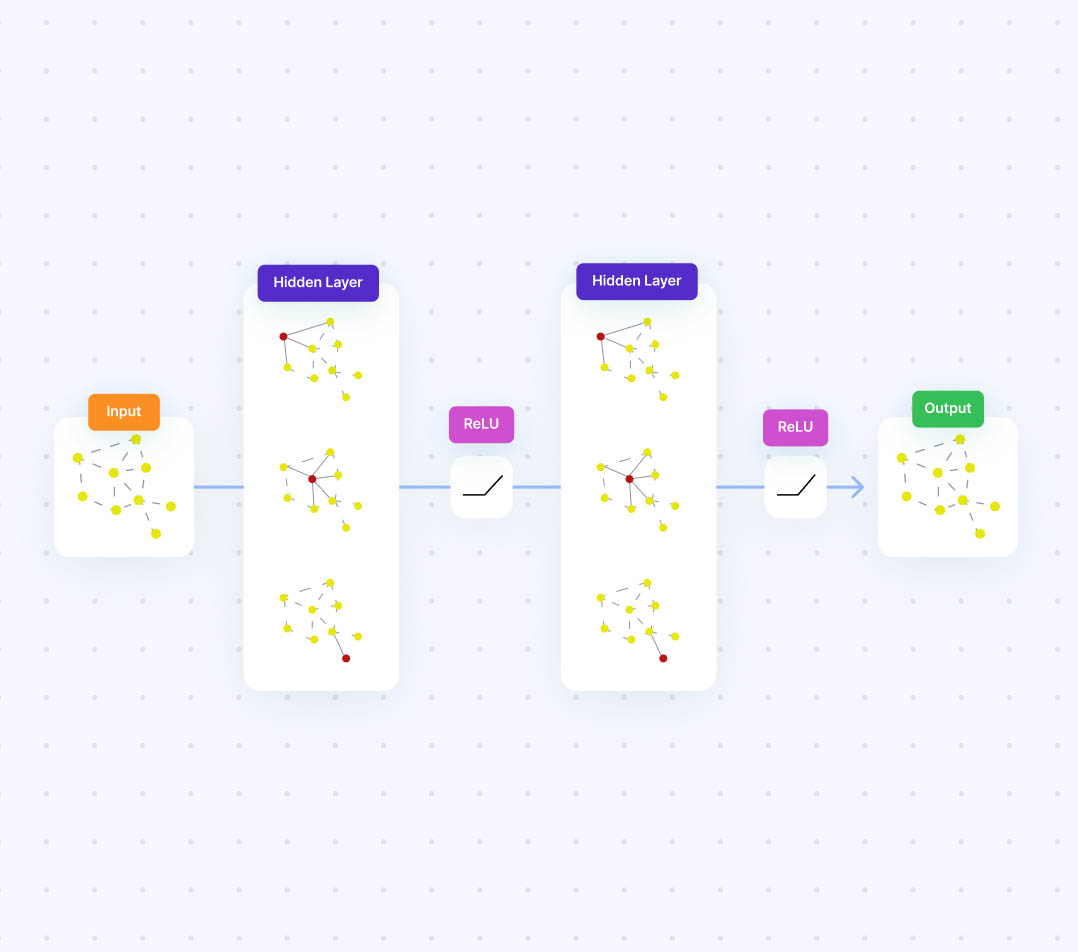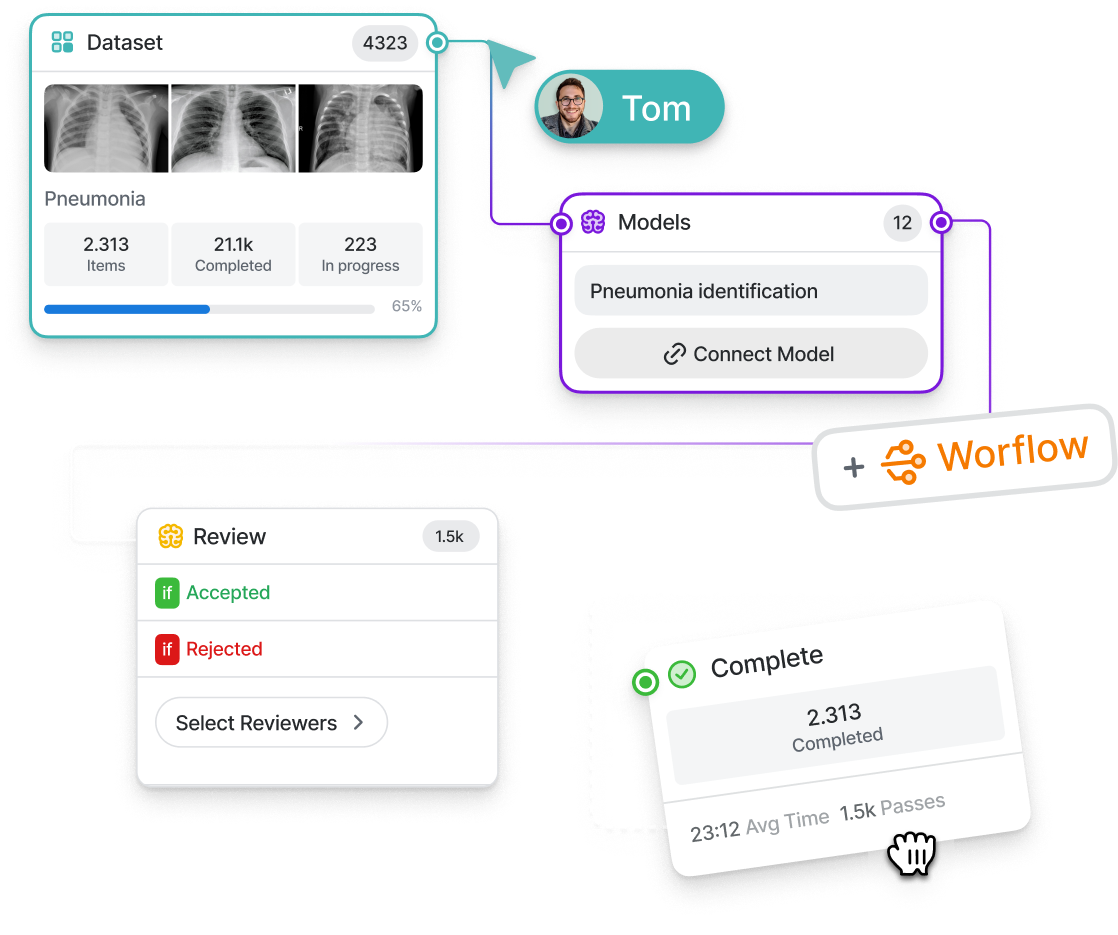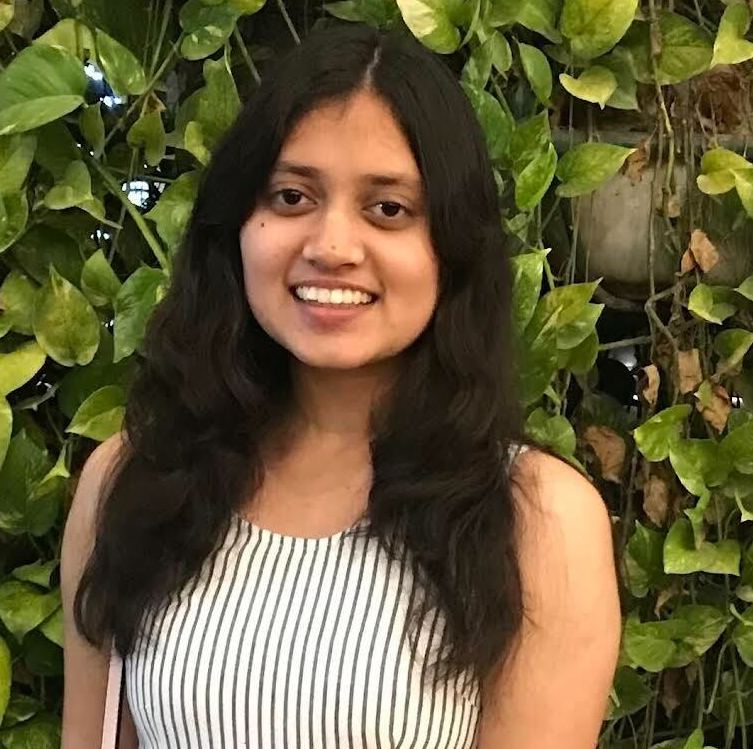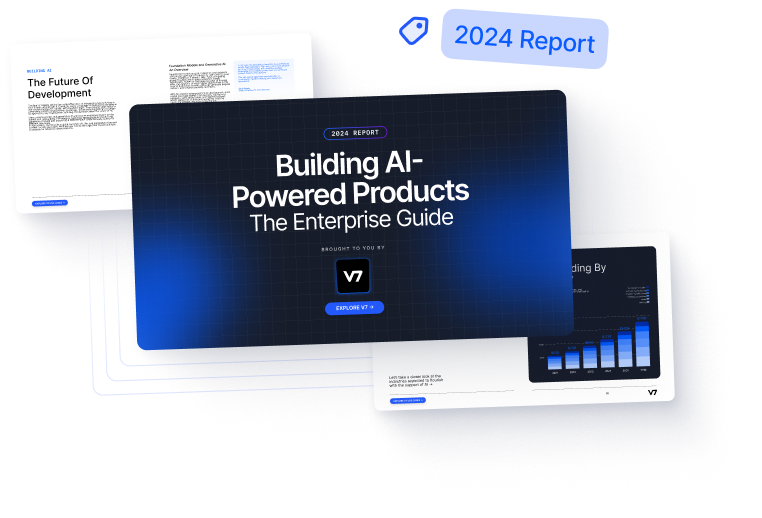# A Beginner’s Guide to Graph Neural Networks

What are Graph Neural Networks (GNN)? Learn more about their architecture, applications in computer vision, and the reasons for their increasing popularity.10
December 9, 2022Graphs are networks that represent relationships between objects through some events. In the real world, graphs are ubiquitous; they can be seen in complex forms such as social networks, biological processes, cybersecurity linkages, fiber optics, and as simple as nature's life cycle.

Since graphs have greater expressivity than images or texts, Graph Neural Network (GNN) applications have increased tremendously in the past decade. They are actively used in drug discovery, human-object interaction, text classification, point cloud classification and segmentation, and so on, all of which are discussed in detail later.

Here’s what we'll cover:

##### 💡 Pro tip: Want some tips on labeling your data? Head over to our Data Annotation Guide

Train ML models and solve any computer vision task faster with V7.

Don't start empty-handed. Explore our repository of 500+ open datasets and test-drive V7's tools.

Ready to streamline AI product deployment right away? Check out:

## What is a Graph?

A graph comprises nodes (objects or entities) and the links that determine relationships between nodes. Mathematically, the nodes are called ‘Vertices,’ and the links are called ‘Edges.’ Graphs can be of two forms—Directed or Undirected

### Directed Graph

Directed graphs denote the direction of dependency between nodes. It can either be uni-directed or bi-directed.

A real-world example of directed graphs can be Followers on Instagram

The above figure shows an example of a unidirectional link: Joan follows Justin Bieber, but Justin Bieber does not follow Joan back. Since Justin Bieber follows Kanye and Kanye follows Justin Bieber back, they are bi-directionally linked.

### Undirected Graph

Undirected graphs do not have any directions of dependencies; the nodes can be considered mutually linked.

A real-world example of undirected graphs can be Followers on LinkedIn.

## Types of Graph Prediction Problems

There are mainly three possible types of graph prediction problems—Graph-level, Node-level, and Edge-level. The basic building block for these graph prediction problems is Graph Convolution. Let’s dive right into it.

### Graph Convolution

Since images comprise pixels, graph nodes can be analogously considered as pixels - each node (pixel) is connected to adjacent nodes (pixels). Convolution in Convolutional Neural Network (CNN) is a simple sliding window method over the whole image that multiplies the image pixels with the filter weights. Similarly, graph convolution uses information from the neighboring nodes to predict features of a given node xi. Convolution is an operation that happens on euclidean data since data in graphs is unordered and dynamic; it is non-euclidean data. Hence, a function needs to be applied to the node’s features to transform them into a latent space hi, which can then be used for further algorithmic computation.

### Graph-level Prediction

A graph-level task involves predicting some property for the complete graph. An example of such a task could be determining whether a social network group comprises ‘friends,’ ‘family,’ or ‘corporates.’ With images, the graph-level prediction would be analogous to image classification by assigning image labels such as ‘rainy,’ ‘sunny,’ or ‘snowy’ to images of different seasons. Similarly, for text, it could mean predicting the context of the text, whether it belongs to the ‘news,’ ‘law,’ or ‘education’ category.

Let’s talk about a specific task under graph-level prediction, i.e., Graph Classification.

#### Graph Classification

All the node features are aggregated to obtain a classification label for the complete graph, and some permutation-invariant (i.e., indifferent to the order of inputs) function such as mean, sum, or pooling is applied to them.

### Node-level Prediction

A node-level task involves predicting some property for the node or the node object itself. Let’s consider an example from chemistry. In a graph containing the biomolecular structure of a substance comprising two compounds, A and B, predicting whether a node (a molecule) belongs to compound A or compound B denotes node-level prediction. In the case of images, this task is analogous to predicting the class of all individual pixels, such as semantic segmentation, whereas, with text, this is similar to predicting parts of speech.

#### Node Classification

To predict a label for an individual node denoted by Zi, the function f is applied to the individual node features hi

### Edge-level Prediction

An edge-level prediction involves predicting the relationship between different node objects. For example, imagine a cricket scene with a batsman, a bowler, a fielder, an umpire, and the audience. In the graph world, all of these can be represented as nodes having some links with each other. Now, predicting the facts that the bowler “is bowling” to the batsman, or a fielder “is catching the ball,” or the audience “is watching” the match is an example of edge-level prediction. This is analogous to action recognition or scene understanding in images and predicting the dependency tree for words in texts.

#### Edge Classification

To predict the labels for edges, the function f is applied over the features aggregated from the nodes connecting the edge as well as the existing edge features if there are any.

## Challenges in Analyzing a Graph

One of the significant challenges in analyzing a graph is the non-euclidean nature of the data.

The size of the graph is dynamic. The number of nodes can range from tens or hundreds to the order of millions; similarly, each node can have a variable number of edges. Due to this property, it is challenging to represent and analyze graphs by existing standard methods for images and texts.

Images and texts have a fixed number of attributes whereas graphs can expand or contract with respect to time. Therefore, representing graphs by an adjacency matrix is inefficient as it can create very sparse matrices. Also, there can be multiple adjacency matrices representing the same graph. They are not permutation invariant as there is no certainty that they will generate the same result. To deal with this problem, adjacency lists can be used to represent graphs as they can handle the problem of sparsity and permutation invariance.

Analyzing graphs is difficult due to their dynamic nature. Standard convolution that is applied on images cannot be applied here. There have been several attempts to modify convolutions to suit the graph data structure.

One is adopting depthwise separable convolutions to handle the dynamic data dimensions.

Another strategy was to use dilated convolutions to increase the receptive field for capturing more features for increasing graph dimensions.

Unfortunately, both these attempts failed because the kernel sizes and dilation rates need to be set up manually depending on the graph, which is undoable in real scenarios. Hence, Graph Neural Networks were proposed to deal with graph prediction problems effectively.

## What is a Graph Neural Network (GNN)?

A Graph Neural Network (GNN) is a ‘Graph In, Graph Out’ network. It takes the input graph comprising embeddings for edges, nodes, and global context and generates the output graph with transformed and updated embeddings by preserving the graph symmetry. GNNs are efficient architectures for solving different graph prediction problems for graph-level, node-level, and edge-level tasks.

## Graph Neural Network Architectures

GNN architectures can mainly be categorized into Spectral, Spatial, and Sampling methods.

Let’s understand each of them and look at some of the most common GNN architectures.

### Spectral Methods

To understand this domain of methods, let’s first briefly understand some graph theory.

Spectral methods perform graph convolution in the spectral domain. Graphs are converted from spatial domain to spectral domain using the concept of discrete Fourier transform. As the graph is projected to an orthogonal space, a feature matrix U will be obtained from a spectral decomposition of a Laplacian matrix. Hence, U is a matrix comprising eigenvalues of corresponding eigenvectors. The graph Fourier transform is obtained by taking a dot product of eigenvalues with a function f that maps the graph vertices to some number on the real line which can ultimately represent as:

Since we have obtained the Fourier transform, graph convolution in the spectral domain is simply a multiplication of the spectral input signal and the spectral convolution kernel.

The above equation represents that convolution operation in the spatial domain changes to the product of the Fourier transform of signal (F1) and kernel (F2) in the spectral domain.

Now that you know what spectral methods are, let's understand the two most common GNN architectures under this category.

#### Spectral Networks (SCNN)

This method replaces the spectral convolutional kernel with a self-learning diagonal matrix. It allows learning the convolutional filters for graph prediction tasks.

The modified convolutional kernel is represented as:

Here, gθ is the set of self-learning parameters. X is an N-dimensional input vector. U is the matrix for eigen vectors, and 𝛬 is the diagonal matrix.

Given the advantage that the kernel is learnable, there are also some significant disadvantages that make this method inefficient.

1. This method would be computationally inefficient for large graphs as the product of U, 𝛬, U^T will need to be calculated during each forward pass.
2. The number of parameters in the kernel depends on the number of vertices in the graph. Hence for large graphs, the method remains inefficient.
3. The filter is applied to the whole graph; hence it will be difficult to obtain local information.

#### Graph Convolutional Networks (GCN)

Graph Convolutional Network (GCN) is one of the most commonly used methods due to its simple, scalable architecture and computational efficiency.

The simplest GCN has three layers:

• Convolutional layer
• Linear layer
• Non-linear activation layer

First, convolution is performed for each node in the convolution layer graph. The feature information from the neighbors of the nodes is aggregated and the nodes are updated likewise. Next, a non-linear activation function such as ReLU is applied to the output of the convolution layer. Similarly, multiple convolutions and non-linear activation layers can be stacked to reach optimal accuracy.

GCNs can perform node-level as well as graph-level prediction tasks. Node-level classification is possible with local output functions which classify individual node features to predict a tag. For graph-level classification, features from the entire graph are aggregated using differentiable pooling, which is then used to predict a label for the complete graph.

While GCNs are easier to code and implement, there are certain limitations accompanying them:

1. GCNs do not support edge features.
2. The notion of message passing is non-existent with GCNs, which restricts its usage to only those cases where all required information is present in the nodes.

Spatial Methods

Spectral methods have the following disadvantages:

1. They are not suitable for undirected graphs.
2. Contrary to the graphs' dynamic nature, the graph structures cannot be updated during training.
3. They are computationally more intensive than spatial methods.

Therefore, let’s look at what spatial methods offer and which are commonly used architectures under this category.

Spatial methods follow the standard approach of graph convolution used under graph topology—transforming the node’s features with a permutation-invariant function and aggregating those features to update the node’s feature values.

#### Message Passing Neural Network (MPNN)

Message-passing phenomena in the graphs are essential to utilize graph connectivity's potential fully. Specific information can be encoded in the edges or nearby nodes relevant to the prediction task. Hence, message passing can supply information to the particular node or edge.

Intuitively, message passing is similar to convolution for images. Convolution operation in images parses over the entire image; likewise, in message passing after k layers, a node will be aware of the information in nodes k steps away from it. Mathematically, a message mij sent across the edges i and j can be defined as:

Here f is a small Multi-Layer Perceptron (MLP).

When messages arrive at nodes, the feature representation of the node will update to contain these messages. All the messages are combined using a permutation-invariant aggregator function such as sum. The messages are combined with the existing feature vectors of the node, and they are passed into another MLP to output the final feature vector of the node.

MPNN is a compelling framework to use as it’s very generic, however, it suffers from scalability issues because it needs to process and store edge features as well as node features.

#### Graph Attention Networks (GAT)

GAT introduces the concept of attention mechanism in graph networks. In typical algorithms, the same convolutional kernel parameters are applied over all nodes of the graph; however, in real scenarios, they can either lead to loss or overestimation of certain information. Therefore, adopting different convolutional parameters would allow adjusting the degree of association between nodes and determining the corresponding importance of nodes.

The attention coefficients are calculated by passing node or edge features into an attention function. The softmax function is applied over the obtained value to give the final weights.

Mathematically, the update rule of GAT is represented as:

Here, 𝛼ijk denotes the attention coefficients, Wk is the weight matrix, and hj is the node features.

Overall, GATs are scalable, computationally efficient, and invariant to the choice of attention function used.

### Sampling Methods

In the real world, graphs are usually complex and contain a massive number of nodes and edges. Because of their dynamic nature, they can even expand to larger sizes. In such scenarios, computing feature vectors of nodes by aggregating features from all the neighboring nodes would be computationally inefficient. Hence, to handle the scalability issues, we will sample and use only a subset of nodes instead of all.

We will look at two of the most exciting sampling methods—GraphSage and DeepWalk.

#### GraphSage

GraphSage employs a basic strategy of uniform sampling. The nodes obtained from uniform sampling will only contribute to feature information. The aggregated feature information from these nodes would finally be used to perform node or graph classification.

GraphSage extends the neighborhood depth k on each layer. The algorithm will learn feature information from k nodes away with every additional layer. This approach saves a lot of computation time as all nodes are not involved in computation for each layer.

Depending on the task, this algorithm is flexible enough to be trained in either a supervised or unsupervised manner. There is also a possibility to train the following aggregation functions alongside—mean aggregator, LSTM aggregator, and max pooling aggregator. This facility will allow the model to learn which aggregator function will aggregate the features better for a specific task.

#### DeepWalk

This algorithm works in two stages. The first corresponds to discovering the local graph structure, and the second involves applying a skip-gram model to the sequences detected in the first stage.

Stage 1: Random Walks

The network is traversed through random walks. To begin with, a node is selected randomly, then out of all its neighboring nodes, and another node is selected randomly. Likewise, it goes on until the sequence length reaches its limit. The number of random walks is given by parameter ‘k,’ and the length of each sequence is denoted by parameter ‘l.’ Increasing the parameter ‘k’ would lead to a broader graph exploration, whereas increasing ‘l’ means distant nodes are also similar.

Stage 2: Skip-gram model

Skip-gram is a popular method used to learn word embeddings. This is used here because the distribution of the words in a corpus and the nodes in a graph both follow the power law. In the context of the text, given a corpus and window size, the words that appear in the same window tend to have similar meanings and closer word embeddings. Likewise, skip-gram attempts to maximize the similarity of the nodes that occur in the same random walk.

Thereafter, a set of random vectors are generated for each node to learn the node embeddings using the skip-gram method. Next, gradient descent is applied to these vectors to update the node embeddings and maximize the probability of the neighboring nodes given a node by using a softmax function. When all walks are covered, further optimization can be continued on the same new random walks that can be generated.

Build ML workflows. Deploy AI faster.

Plot the best routes for your training data with 8 workflow stages to arrange, connect, and loop any way you need.## Applications of Graph Neural Networks

Let’s go through a few most common uses of Graph Neural Networks.

### Point Cloud Classification and Segmentation

LiDAR sensors are prevalent because of their applications in environment perception, for example, in self-driving cars. They plot the real-world data in 3D point clouds used for 3D segmentation tasks. GNNs can be used to represent these points as a graph and classify them for segmentation. The figure below shows an example of how a GNN model converts the point clouds to give classification tags.

### Text Classification

A corpus of words can be represented in the form of a graph, having nodes as words and edges as the connections between them. Classification can be performed on the node level or graph level. Text classification with GNNs can be used for real-world applications such as news categorization, product recommendation, or disease detection from symptoms. GNNs provides the advantage of learning about long-distance semantic relationships and give a more precise visualization of the interdependencies of words in a text.

### Human-Object Interaction

Since graphs consist of links (edges) between objects (nodes) they are a great way to represent interactions between them. In a scene, humans and objects can be modeled as nodes, and their relationship (represented by edges) can be identified using edge prediction. In computer vision, GNNs can be used to solve the problem of Human Activity Recognition.

### Relation Extraction in NLP

GNNs can be used to extract the relations between words in NLP. It refers to predicting the text's semantic relations, which could be contextual or grammatical, such as establishing a dependency tree. The figure below shows a GNN architecture for the specific task of dialogue relation extraction.

### Physics

GNNs is an active field of research in the domain of Particle Physics. It deals with studying laws related to particle interactions which can be very well represented with graphs. For example, GNNs can be used to predict the system properties of collision dynamics. Currently, GNNs are being used at the Large Hadron Collider to detect interesting particles from the images produced by various particle physics experiments.

### Chemistry (Drug Discovery)

Drug discovery is one of the most pressing challenges in chemistry and society. The fact that billions of dollars are invested annually in research for drug discovery, and once begun, it takes nearly 10-12 years for the drug to be released to the public calls for some automation. AI using GNNs can help reduce the time taken for research and assist in feedback or screening processes. GNNs can address questions such as ‘Is X drug safe?’, ‘What are the possible combinations of bonds to generate a drug Y similar to X?’, ‘Which drug can be used to treat disease Z?’ and so on.

The given figure shows the role of GNN in inferring a question about a drug based on the existing relational data between drugs and diseases.

## GNN in Computer Vision: Key Takeaways

• GNNs are inspired by graph theory and convolutional neural nets. They take the raw real-world data modeled in the graph as input and generate an output graph with either node, edge, or global predictions.
• GNN approaches can be broadly divided into three main categories: Spectral, Spatial, and Sampling.
• Spectral methods transform the input graph to a spectral (frequency) domain using the graph fourier transform for further processing. Some examples are Spectral Networks (SCNN) and Graph Convolutional Networks (GCN).
• Spatial methods are computationally less expensive than spectral methods. These can work on any kind of graph - directed or undirected and are flexible enough to adopt updations during training. Message Passing Neural Networks (MPNN) and Graph Attention Networks (GAT) are common architectures here.
• Sampling methods take into account the challenge of scalability for spectral and spatial methods. They sample a subset of nodes through different techniques. GraphSage performs uniform node sampling, whereas DeepWalk generates random node sequences.
• GNNs in Computer Vision have diverse applications across the industry. While they are actively researched for tasks such as Traffic Prediction, Social Network Analysis, Drug Discovery, and many more, they still hold a strong potential to expand to new domains due to their inherently dynamic and scalable structure.

What is Data Labeling and How To Do It Efficiently [Tutorial]

Train Test Validation Split: How To & Best Practices

An Introductory Guide to Quality Training Data for Machine Learning

27+ Most Popular Computer Vision Applications and Use Cases

Supervised vs. Unsupervised Learning [Differences & Examples]Yesha is a master’s student at Université de Montréal pursuing Computer Science with Machine Learning. She is passionate about exploring AI techniques that benefit society and sharing her learnings with the AI community.

“Collecting user feedback and using human-in-the-loop methods for quality control are crucial for improving Al models over time and ensuring their reliability and safety. Capturing data on the inputs, outputs, user actions, and corrections can help filter and refine the dataset for fine-tuning and developing secure ML solutions.”Name
Company
GUIDE
Building AI-Powered Products: The Enterprise Guide

Building AI products? This guide breaks down the A to Z of delivering an AI success story.

Oops! Something went wrong while submitting the form.## Related articles

Gain control of your training data
15,000+ ML engineers can’t be wrongGUIDE
FREE
Building AI-Powered Products: The Enterprise Guide
A Comprehensive Guide

Building AI products? This guide breaks down the A to Z of delivering an AI success story.